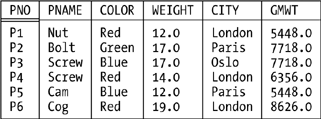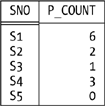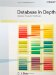# Extend and Summarize

As you might have noticed, the algebra as I've described it so far has no conventional computational capabilities. Now, SQL does; for example, we can write queries along the lines of SELECT A+B AS . . . or SELECT SUM(C) AS . . . (and so on). However, as soon as we write that "+" or that SUM, we've gone beyond the bounds of the relational algebra as originally defined. So we need to add some new operators to the algebra in order to provide this kind of functionality. That's what extend and summarize are for. Loosely, extend supports computation "across tuples," and summarize supports computation "down attributes." Let's take a closer look.

#### Extend

I'll start with an example, since this operator might be new to you. Suppose part weights are given in pounds, and we want to see those weights in grams. There are 454 grams to a pound, and so we can write:

`   EXTEND P ADD              | SELECT P.*,   ( WEIGHT * 454 AS GMWT )  |    ( P.WEIGHT * 454 ) AS GMWT                             | FROM   P `

Given our usual sample values, the result looks like this:NOTE

Important: Relvar P is not changed in the database! EXTEND is not an SQL-style ALTER TABLE; the EXTEND expression is just an expression, and like any expression it simply denotes a value.

To continue with the example, consider now the query "Get part number and gram weight for parts with gram weight greater than 7000 grams." Here's a Tutorial D formulation:

`   ( ( EXTEND P        ADD ( WEIGHT * 454 AS GMWT ) )          WHERE GMWT > 7000.0 ) { PNO, GMWT } `

SQL analog:

`   SELECT P.*, ( P.WEIGHT * 454 ) AS GMWT   FROM   P   WHERE  ( P.WEIGHT * 454 ) > 7000.0 `

As you can see, the expression P.WEIGHT * 454 appears twice in the SQL formulation, and we have to hope the implementation will be smart enough to recognize that it need evaluate that expression just once per tuple instead of twice. In Tutorial D, by contrast, the expression appears only once.

The problem this example illustrates is that SQL's SELECT - FROM - WHERE template is simply too rigid. What we need to do, as the Tutorial D formulation makes clear, is perform a restriction of an extension; in SQL terms, we need to apply the WHERE clause to the result of the SELECT clause, as it were. But the SELECT - FROM - WHERE template forces the WHERE clause to apply to the result of the FROM clause, not the SELECT clause. To put it another way: in many respects, it's the whole point of the algebra that (thanks to closure) relational operations can be combined in arbitrary ways; but SQL's SELECT - FROM - WHERE template effectively means that queries must be expressed as a cartesian product,[*] followed by a restrict, followed by some combination of project and/or extend and/or rename and many queries just don't fit this pattern.

[*] It's true thanks to, among other things, the ability to nest subqueries in the FROM clause that the operands to that cartesian product aren't limited to being named tables (base tables or views), but the FROM clause as such still basically means cartesian product.

Incidentally, you might be wondering why I didn't formulate the SQL query like this:

`   SELECT P.*, ( P.WEIGHT * 454 ) AS GMWT   FROM   P   WHERE  GMWT > 7000.0 `

(The change is in the last line.) The reason is that GMWT is the name of a column of the final result; table P has no such column, the WHERE clause thus makes no sense, and the expression fails on a syntax error.

Actually, the SQL standard does allow the query under discussion to be formulated in a style that's a little closer to that of Tutorial D:

`   SELECT TEMP.PNO, TEMP.GMWT   FROM ( SELECT P.PNO, ( P.WEIGHT * 454 ) AS GMWT          FROM P ) AS TEMP   WHERE  TEMP.GMWT > 7000.0 `

As noted earlier, however, not all SQL products allow nested subqueries to appear in the FROM clause in this manner.

Here now is a definition. Let r be a relation. Then the extension:

`   EXTEND r ADD ( exp AS X ) `

is a relation with (a) heading equal to the heading of r extended with attribute X, and (b) body consisting of all tuples t such that t is a tuple of r extended with a value for attribute X that is computed by evaluating exp on that tuple of r. Relation r must not have an attribute called X, and exp must not refer to X. Observe that the result has cardinality equal to that of r and degree equal to that of r plus one. The type of X in that result is the type of exp.

#### Summarize

Again I'll start with an example (the query is "For each supplier, get the supplier number and a count of the number of parts that supplier supplies"):

`   SUMMARIZE SP PER ( S { SNO } ) ADD ( COUNT ( ) AS P_COUNT ) `

Given our usual sample values, the result looks like this:Note the tuple for supplier S5 in particular; the PER specification indicates that the summarizing is to be done "per the projection of S on SNO," which means it produces a result with five tuples (one for each tuple in that projection). By contrast, the following SQL expression (which might be thought to be equivalent to the Tutorial D formulation):

`   SELECT SP.SNO, COUNT(*) AS P_COUNT   FROM   SP   GROUP  BY SP.SNO `

produces a result containing tuples for suppliers S1, S2, S3, and S4 only. The reason is, of course, that it extracts supplier numbers from SP, and supplier S5 doesn't appear in SP at all. An SQL expression that is equivalent to the Tutorial D formulation is:

`   SELECT S.SNO, TEMP.P_COUNT   FROM   S, LATERAL ( SELECT COUNT(*) AS P_COUNT             FROM   SP             WHERE  SP.SNO = S.SNO ) AS TEMP `

As I've already pointed out, however, not all SQL products support this kind of expression.

NOTE

The standard requires the keyword LATERAL here because the subquery refers "laterally" to another element in the same FROM clause.

Here now is the definition. Let r and s be relations such that s is of the same type as some projection of r, and let the attributes of s be A1, A2, . . ., An. Then the summarization:

`   SUMMARIZE r PER ( s ) ADD ( summary AS X ) `

is a relation with (a) heading equal to the heading of s extended with attribute X, and (b) body consisting of all tuples t such that t is a tuple of s extended with a value for attribute X. That X value is computed by evaluating summary over all tuples of r that have the same value for attributes A1, A2, . . . , An as tuple t does. Relation s must not have an attribute called X, and summary must not refer to X. Observe that the result has cardinality equal to that of s and degree equal to that of s plus one. The type of X in that result is the type of summary.

What "summaries" are supported? Well, the set is open-ended but certainly includes the usual COUNT, SUM, AVG, MAX, and MIN. Here's an example involving MAX and MIN:

`   SUMMARIZE SP PER ( SP { SNO } ) ADD ( MAX ( QTY ) AS MAXQ ,                                         MIN ( QTY ) AS MINQ ) `

This example illustrates two further points:

• It's possible to perform "multiple summarizations" within a single SUMMARIZE. (I didn't mention the point earlier, but analogous remarks apply to RENAME and EXTEND as well.)

• The PER operand in this example isn't just "of the same type as" some projection of the relation to be summarized, it actually is such a projection. In such cases the expression can be simplified slightly, as here:

`   SUMMARIZE SP BY { SNO } ADD ( MAX ( QTY ) AS MAXQ ,                                 MIN ( QTY ) AS MINQ ) `

Other legal "summaries" include COUNTD, SUMD, and AVGD (where "D" stands for "distinct" and means "eliminate redundant duplicate values before summarizing"); AND, OR, and XOR (for attributes of type BOOLEAN); INTERSECT, UNION, and D_UNION (for relation-valued attributes); and so on.

By the way, COUNT and the rest here are not aggregate operators, though most of them do have the same names as aggregate operators (SQL confuses these two notions, with unfortunate results). An aggregate operator invocation is a scalar expression, and it returns a scalar value.[*] Here's an example:

[*] Nonscalar aggregate operators can be defined too, but they're beyond the scope of this book.

`   VAR N INTEGER ;   N := COUNT ( S WHERE CITY = 'London' ) ; `

Summaries, by contrast, are merely operands to SUMMARIZE invocations; they have no meaning outside that context, and in fact can't be used outside that context.

Here's one more SUMMARIZE example ("How many suppliers are there in London?"):

`   SUMMARIZE ( S WHERE CITY = 'London' ) ADD ( COUNT ( ) AS N ) `

Again, this example illustrates two points:

• This SUMMARIZE has no PER specification. By default, therefore, the summarizing is done per TABLE_DEE that is, the expression shown is shorthand for:

`   SUMMARIZE ( S WHERE CITY = 'London' )             PER ( TABLE_DEE ) ADD ( COUNT ( ) AS N ) `

I remind you again that TABLE_DEE is the relation that has no attributes and one tuple (and is thus certainly of the same type as "some projection of" every possible relation! namely, the projection of the relation in question on the empty set of attributes). The output from this SUMMARIZE therefore has one attribute and one tuple, and given our usual sample data values it looks like this:[*]

[*] The fact that the picture involves no double underlining is not a mistake. See Exercise 4-28 in Chapter 4.• I said a moment ago that aggregate operator invocations and summaries were different things, and you might be wondering what the difference is between the SUMMARIZE example under discussion and the COUNT operator invocation we saw a few paragraphs back:

`   COUNT ( S WHERE CITY = 'London' ) `

The overriding difference is, of course, that SUMMARIZE returns a relation and aggregate operators return a scalar. For further discussion, see Exercise 5-11 at the end of this chapter.Database in Depth: Relational Theory for Practitioners
ISBN: 0596100124
EAN: 2147483647
Year: 2006
Pages: 127
Authors: C.J. Date

Similar book on Amazon